Saturday, July 11, 2020
Home > CBSE Class 12 > NCERT Solutions Class 12 Maths Three Dimensional Geometry Exercise 11.3

# NCERT Solutions Class 12 Maths Three Dimensional Geometry Exercise 11.3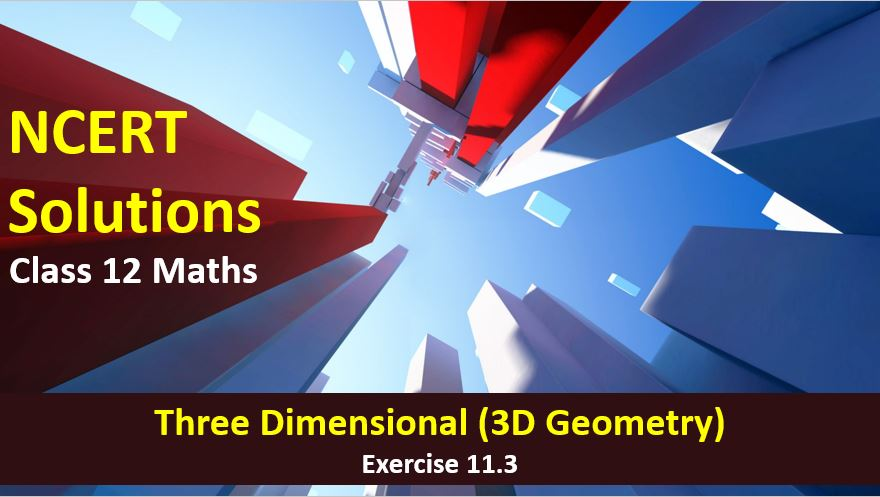# NCERT Solutions for Class 12 Maths Three Dimensional Geometry

Hi Students, Welcome to Amans Maths Blogs (AMB). In this post, you will get the NCERT Solutions for Class 12 Maths Three Dimensional Geometry Exercise 11.3.

As we know that all the schools affiliated from CBSE follow the NCERT books for all subjects. You can check the CBSE NCERT Syllabus. Thus, NCERT Solutions helps the students to solve the exercise questions as given in NCERT Books.

NCERT Solutions for class 12 is highly recommended by the experienced teacher for students who are going to appear in CBSE Class 12 and JEE Mains and Advanced and NEET level exams. Here You will get NCERT Solutions for Class 12 Maths 3D Geometry Exercise 11.3 of all questions given in NCERT textbooks of class 12 in details with step by step process.

The PDF books of NCERT Solutions for Class 12 are the first step towards the learning and understanding the each sections of Maths, Physics, Chemistry, Biology as it all help in engineering medical entrance exams. To solve it, you just need to click on download links of NCERT solutions for class 12.

CBSE Class 12th is an important school class in your life as you take some serious decision about your career. And out of all subjects, Maths is an important and core subjects. So CBSE NCERT Solutions for Class 12th Maths is major role in your exam preparation as it has detailed chapter wise solutions for all exercise. This NCERT Solutions can be downloaded in PDF file. The downloading link is given at last.

NCERT Solutions for Class 12 Maths are not only the solutions of Maths exercise but it builds your foundation of other important subjects. Getting knowledge of depth concept of CBSE Class 12th Maths like Algebra, Calculus, Trigonometry, Coordinate Geometry help you to understand the concept of Physics and Physical Chemistry.

## NCERT Solutions for Class 12 Maths Three Dimensional Geometry

NCERT Solutions for Class 12 Maths Three Dimensional Geometry Exercise 11.3 Ques No 1.

In each of the following cases, determine the direction cosines of the normal to the plane and the distance from the origin.

(a) z = 2

NCERT Solutions:

If the Cartesian equation of plane is Ax + By + Cz = D, then the direction ratios of the normal N = Ai + Bj + Ck are (A, B, C). Hence, its direction cosines are.

Given that the equation of plane is z = 2 ⇒ 0.x + 0.y + 1.z = 2. Then, N = 0i + 0j + 1k. Hence, the direction cosines are (0, 0, 1).

Now, we know that the perpendicular from P(x1, y1, z1) to the plane is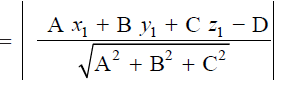Thus, the perpendicular from origin O(0, 0, 0) to the plane is.

(b) x + y + z = 1

NCERT Solutions:

If the Cartesian equation of plane is Ax + By + Cz = D, then the direction ratios of the normal N = Ai + Bj + Ck are (A, B, C). Hence, its direction cosines are.

Given that the equation of plane is x + y + z = 1. Then, N = i + j + k and the direction ratios are (1, 1, 1). Thus, the direction cosine of the normal to plane is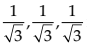.

Now, we know that the perpendicular from P(x1, y1, z1) to the plane isThus, the perpendicular from origin O(0, 0, 0) to the plane is.

(c) 2x + 3y – z = 5

NCERT Solutions:

If the Cartesian equation of plane is Ax + By + Cz = D, then the direction ratios of the normal N = Ai + Bj + Ck are (A, B, C). Hence, its direction cosines are.

Given that the equation of plane is 2x + 3y – z = 5. Then, N = 2i + 3jk and the direction ratios are (2, 3, –1). Thus, the direction cosine of the normal to plane is.

Now, we know that the perpendicular from P(x1, y1, z1) to the plane isThus, the perpendicular from origin O(0, 0, 0) to the plane is.

(d) 5y + 8 = 0

NCERT Solutions:

If the Cartesian equation of plane is Ax + By + Cz = D, then the direction ratios of the normal N = Ai + Bj + Ck are (A, B, C). Hence, its direction cosines are.

Given that the equation of plane is 5y + 8 = 0 ⇒ 0.x + 5y + 0.z = –8. Then, N = 0i + 5j + 0k and the direction ratios are (0, 5, 0). Thus, the direction cosine of the normal to plane is (0, 1, 0).

Now, we know that the perpendicular from P(x1, y1, z1) to the plane isThus, the perpendicular from origin O(0, 0, 0) to the plane is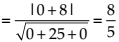.

NCERT Solutions for Class 12 Maths Three Dimensional Geometry Exercise 11.3 Ques No 2.

Find the vector equation of a plane which is at a distance of 7 units from the origin and normal to the vector 3i + 5j − 6k.

NCERT Solutions:

Given that the normal vector is N = 3i + 5j − 6k.

Then,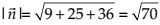and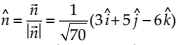.

Hence, the required equation of the plane is.

NCERT Solutions for Class 12 Maths Three Dimensional Geometry Exercise 11.3 Ques No 3.

Find the Cartesian equation of the following planes:

(a) r ⋅ (i + jk) = 2

NCERT Solutions:

To find the Cartesian equation of plane, put r = xi + yj + zk.

Thus, (xi + yj + zk) ⋅ (i + jk) = 2

⇒ x + y − z = 2

(b) r ⋅ (2i + 3j − 4k) = 1

NCERT Solutions:

To find the Cartesian equation of plane, put r = xi + yj + zk.

Thus, (xi + yj + zk) ⋅ (2i + 3j − 4k) = 1

⇒ 2x + 3y − 4z = 1

(c) r ⋅ [(s − 2t)i + (3 − t)j + (2s + t)k] = 15

NCERT Solutions:

To find the Cartesian equation of plane, put r = xi + yj + zk.

Thus, (xi + yj + zk) ⋅ [(s − 2t)i + (3 − t)j + (2s + t)k] = 15

(s − 2t)x + (3 − t)y + (2s + t)z = 1

NCERT Solutions for Class 12 Maths Three Dimensional Geometry Exercise 11.3 Ques No 4.

In the following cases, find the coordinates of the foot of the perpendicular drawn from the origin.

(a) 2x + 3y + 4z – 12 = 0

NCERT Solutions: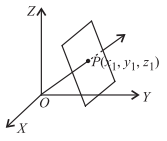Let the coordinate of the foot of the perpendicular P from the origin to the plane be (x1, y1, z1).

Then, the direction ratios of the line OP are x1, y1, z1.

Given equation of the plane in the normal form is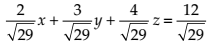whereis the direction cosine of OP.

We know that the direction cosines and direction ratios are proportional. Then,Substituting these values in the given equation of the plane, we get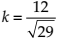.

Thus,the required coordinate of the foot of the perpendicular is (24/29, 36/29, 48/29).

(b) 3y + 4z – 6 = 0

NCERT Solutions:Let the coordinate of the foot of the perpendicular P from the origin to the plane be (x1, y1, z1).

Then, the direction ratios of the line OP are x1, y1, z1.

Given equation of the plane in the normal form is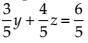where 3/5 and 4/5 are the direction cosine of OP.

We know that the direction cosines and direction ratios are proportional. Then,Substituting these values in the given equation of the plane, we get

k = 6/5.

Thus,the required coordinate of the foot of the perpendicular is (0, 18/25, 24/25).

(c) x + y + z = 1

NCERT Solutions:Let the coordinate of the foot of the perpendicular P from the origin to the plane be (x1, y1, z1).

Then, the direction ratios of the line OP are x1, y1, z1.

Given equation of the plane in the normal form iswhereare the direction cosine of OP.

We know that the direction cosines and direction ratios are proportional. Then,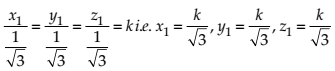Substituting these values in the given equation of the plane, we getThus,the required coordinate of the foot of the perpendicular is (1/3, 1/3, 1/3).

(d) 5y + 8 = 0

NCERT Solutions:Let the coordinate of the foot of the perpendicular P from the origin to the plane be (x1, y1, z1).

Then, the direction ratios of the line OP are x1, y1, z1.

Given equation of the plane in the normal form is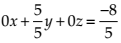where 0, 1, 0 are the direction cosine of OP.

We know that the direction cosines and direction ratios are proportional. Then,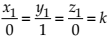or x1 = 0, y1 = k,  z1 = 0.

Substituting these values in the given equation of the plane, we get k = − 8/5

Thus,the required coordinate of the foot of the perpendicular is (0, −8/5, 0).

NCERT Solutions for Class 12 Maths Three Dimensional Geometry Exercise 11.3 Ques No 5.

Find the vector and cartesian equations of the planes

(a) that passes through the point (1, 0, – 2) and the normal to the plane is  i + jk.

NCERT Solutions:

Given plane passes through the point P(1, 0, – 2) and its normal is i + j k.

Thus, we have a = i – 2j and n = i + jk.

Now, the vector equation of the plane passing through a and normal to n is (r – a).n = 0.

Thus, the vector equation of the given plane is [r – (i – 2j)].(i + jk) = 0.

Again the plane passes through the point P(1, 0, – 2) and its normal is i + j k.

We have x1 = 1, y1 = 0, z1 = –2 and the direction cosine is a = 1, b = 1 and c = –2.

Now, the Cartesian equation of the plane passing through (x1, y1, z1) and its direction cosines of the normal (a, b, c) is a(x – x1) + b(y – y1) + c(z – z1) = 0.

Thus, the Cartesian equation of the given plane is

(1)(x – 1) + (1)(y – 0) + (–2)(z – (–2)) = 0 ⇒ x + y – z = 3

(b) that passes through the point (1, 4, 6) and the normal to the plane is  i − 2j + k.

NCERT Solutions:

Given plane passes through the point P(1, 4, 6) and its normal is i − 2j + k.

Thus, we have a = i + 4j + 6k and n = i − 2j + k.

Now, the vector equation of the plane passing through a and normal to n is (r – a).n = 0.

Thus, the vector equation of the given plane is [r – (i + 4j + 6k)].(i − 2j + k) = 0.

Again the plane passes through the point P(1, 4, 6) and its normal is i − 2j + k.

We have x1 = 1, y1 = 4, z1 = 6 and the direction cosine is a = 1, b = –2 and c = 1.

Now, the Cartesian equation of the plane passing through (x1, y1, z1) and its direction cosines of the normal (a, b, c) is a(x – x1) + b(y – y1) + c(z – z1) = 0.

Thus, the Cartesian equation of the given plane is

(1)(x – 1) + (–2)(y – 4) + (1)(z – 6) = 0 ⇒ x – 2y + z + 1 = 0.

NCERT Solutions for Class 12 Maths Three Dimensional Geometry Exercise 11.3 Ques No 6.

Find the equations of the planes that passes through three points.

(a) (1, 1, – 1), (6, 4, – 5), (– 4, – 2, 3)

NCERT Solutions:

The equation of the plane passing through three non collinear points (x1, y1, z1), (x2, y2, z2) and (x3, y3, z3) isThe equation of the plane passing through the given points A(1, 1, – 1), B(6, 4, – 5), C(– 4, – 2, 3) is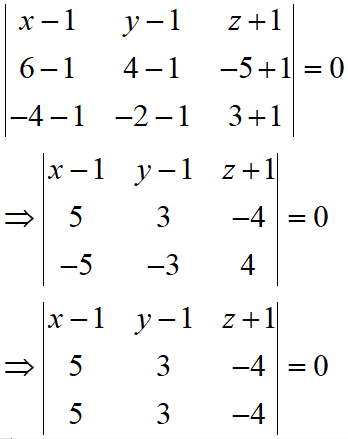Since the two rows of the determinants is same, then the given points are collinear. hence, there are infinite planes passing through the given collinear points.

(b) (1, 1, 0), (1, 2, 1), (– 2, 2, – 1)

NCERT Solutions:

The equation of the plane passing through three non collinear points (x1, y1, z1), (x2, y2, z2) and (x3, y3, z3) isThe equation of the plane passing through the given points A(1, 1, 0), B(1, 2, 1), C(– 2, 2, – 1) is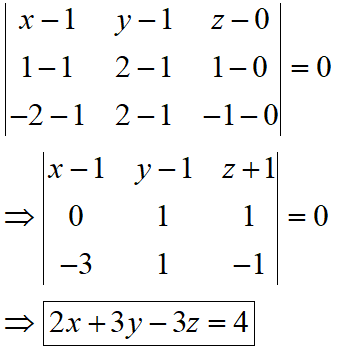NCERT Solutions for Class 12 Maths Three Dimensional Geometry Exercise 11.3 Ques No 7.

Find the intercepts cut off by the plane 2x + y – z = 5.

NCERT Solutions:

Given equation of the plane is 2x + y – z = 5.

In normal of the equation, we haveOn comparing this equation with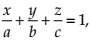we get the intercept on the axes are

a = 5/2, b = 5 and c = -5.

NCERT Solutions for Class 12 Maths Three Dimensional Geometry Exercise 11.3 Ques No 8.

Find the equation of the plane with intercept 3 on the y-axis and parallel to ZOX plane.

NCERT Solutions:

Since the plane is parallel to ZOX plane and its intercept on y-axis is 3, then the plane passes through P(0, 3, 0) and the direction cosine of the normal to the plane is (0, 1, 0).

We have (x1, y1, z1) = (0, 3, 0) and (a, b, c) = (0, 1, 0).

We know that the Cartesian equation of the plane passing through (x1, y1, z1) and its direction cosines of the normal (a, b, c) is a(x – x1) + b(y – y1) + c(z – z1) = 0.

Thus, the equation of the given plane is 0(x – 0) + 1(y – 3) + 0(z – 0) = 0, or y = 3.

NCERT Solutions for Class 12 Maths Three Dimensional Geometry Exercise 11.3 Ques No 9.

Find the equation of the plane through the intersection of the planes 3x – y + 2z – 4 = 0 and x + y + z – 2 = 0 and the point (2, 2, 1).

NCERT Solutions:

The equations of the given planes are

3x – y + 2z – 4 = 0              …(1)

x + y + z – 2 = 0                  …(2)

Now the equation of the plane passing through the intersection of the planes (1) and (2) is

(3x – y + 2z – 4) + k(x + y + z – 2) = 0          …(3)

This plane passes through the point (2, 2, 1). Put x = 2, y = 2 and z = 1.

(6 – 2 + 2 – 4) + k(2 + 2 + 1 – 2) = 0

⇒ k = -2/3

Put k = -2/3 in the equation (3), we get required equation of the given plane

(3x – y + 2z – 4) + (-2/3)(x + y + z – 2) = 0

⇒ 7x – 5y + 4z – 8 = 0

NCERT Solutions for Class 12 Maths Three Dimensional Geometry Exercise 11.3 Ques No 10.

Find the vector equation of the plane passing through the intersection of the planes r.(2i + 2j − 3k) = 7 and r.(2i + 5j + 3k ) = 9 and through the point (2, 1, 3).

NCERT Solutions:

The equations of the given planes are r.(2i + 2j − 3k) = 7 and r.(2i + 5j + 3k ) = 9.

For Cartesian equation of plane, put r = (xi + yj + zk).

(xi + yj + zk).(2i + 2j − 3k) = 7 ⇒ 2x + 2y – 3z – 7 = 0              …(1)

(xi + yj + zk).(2i + 5j + 3k ) = 9 ⇒ 2x + 5y + 3z – 9 = 0             …(2)

Now the equation of the plane passing through the intersection of the planes (1) and (2) is

(2x + 2y – 3z – 7) + k(2x + 5y + 3z – 9) = 0          …(3)

This plane passes through the point (2, 1, 3). Put x = 2, y = 1 and z = 3.

(4 + 2 – 9 – 7) + k(4 + 5 + 9 – 9) = 0 ⇒ k = 10/9.

Put k = 10/9 in the equation (3), we get required equation of the given plane

(2x + 2y – 3z – 7) + (10/9)(2x + 5y + 3z – 9) = 0

⇒ 38x + 68y + 3z – 153 = 0

⇒ (xi + yj + zk).(38i + 68j + 3k) = 153

r.(38i + 68j + 3k) = 153

NCERT Solutions for Class 12 Maths Three Dimensional Geometry Exercise 11.3 Ques No 11.

Find the equation of the plane through the line of intersection of the planes x + y + z = 1 and 2x + 3y + 4z = 5 which is perpendicular to the plane x – y + z = 0.

NCERT Solutions:

The equations of the given planes are

x + y + z – 1 = 0                      …(1)

2x + 3y + 4z – 5 = 0                …(2)

Now the equation of the plane passing through the intersection of the planes (1) and (2) is

(x + y + z – 1) + k(2x + 3y + 4z – 5) = 0

⇒ (1 + 2k)x + (1 + 3k)y + (1 + 4k)z – (1 + 5k) = 0       …(3)

Since the equation of the plane (3) is perpendicular to the plane x – y + z = 0, then

⇒ (1 + 2k)(1) + (1 + 3k)(–1) + (1 + 4k)(1) = 0

⇒ k = –1/3

Put k = –1/3 in the equation (3), we get required equation of the given plane⇒ x – z + 2 = 0

NCERT Solutions for Class 12 Maths Three Dimensional Geometry Exercise 11.3 Ques No 12.

Find the angle between the planes whose vector equations are r ⋅ (2i + 2j − 3k) = 5 and r ⋅ (3i − 3j + 5k) = 3.

NCERT Solutions:

If θ is the angle between the planes r.n1 = d1 and r.n2 = d2, thenGiven that n1 = (2i + 2j − 3k) and n2 = (3i − 3j + 5k). Then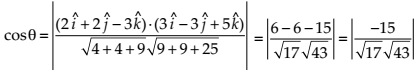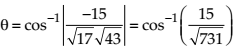NCERT Solutions for Class 12 Maths Three Dimensional Geometry Exercise 11.3 Ques No 13.

In the following cases, determine whether the given planes are parallel or perpendicular, and in case they are neither, find the angles between them.

(a) 7x + 5y + 6z + 30 = 0 and 3x – y – 10z + 4 = 0

NCERT Solutions:

Given equations of planes are 7x + 5y + 6z + 30 = 0 and 3x – y – 10z + 4 = 0.

Since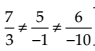, both planes are not parallel.

Since (7)(3) + (5)(–1) + (6)(–10) ≠ 0, both planes are not perpendicular.

If θ is the angle between the given planes, then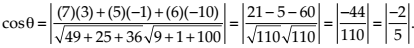(b) 2x + y + 3z – 2 = 0 and x – 2y + 5 = 0

NCERT Solutions:

Given equations of planes are 2x + y + 3z – 2 = 0 and x – 2y + 5 = 0.

Since (2)(1) + (1)(–2) + (3)(0) = 2 – 2 = 0, both planes are perpendicular.

(c) 7x + 5y + 6z + 30 = 0 and 3x – y – 10z + 4 = 0

NCERT Solutions:

Given equations of planes are 2x – 2y + 4z + 5 = 0 and 3x – 3y + 6z – 1 = 0

Since, both planes are parallel.

(d) 2x – y + 3z – 1 = 0 and 2x – y + 3z + 3 = 0

NCERT Solutions:

Given equations of planes are 2x – y + 3z – 1 = 0 and 2x – y + 3z + 3 = 0.

Since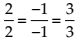, both planes are parallel.

(e) 4x + 8y + z – 8 = 0 and y + z – 4 = 0

NCERT Solutions:

Given equations of planes are 4x + 8y + z – 8 = 0 and y + z – 4 = 0

Since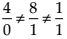, both planes are not parallel.

Since (4)(0) + (8)(1) + (1)(1) ≠ 0, both planes are not perpendicular.

If θ is the angle between the given planes, thenNCERT Solutions for Class 12 Maths Three Dimensional Geometry Exercise 11.3 Ques No 14.

In the following cases, find the distance of each of the given points from the corresponding given plane.

(a) Coordinate of Point is (0, 0, 0) and Equation of Plane is 3x – 4y + 12z = 3.

NCERT Solutions:

Let P(x1, y1, z1) be the given point and Ax + By + Cz = D be the Cartesian equation of the given plane. Then, the perpendicular from P to the plane isThus, the perpendicular distance from the given point P(0, 0, 0) to the plane 3x – 4y + 12 z = 3 is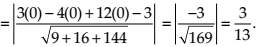(b) Coordinate of Point is (3, – 2, 1) and Equation of Plane is 2x – y + 2z + 3 = 0.

NCERT Solutions:

Let P(x1, y1, z1) be the given point and Ax + By + Cz = D be the Cartesian equation of the given plane. Then, the perpendicular from P to the plane isThus, the perpendicular distance from the given point P(3, – 2, 1) to the plane 2x – y + 2z + 3 = 0 is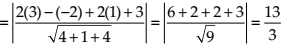(c) Coordinate of Point is (2, 3, – 5) and Equation of Plane is x + 2y – 2z = 9

NCERT Solutions:

Let P(x1, y1, z1) be the given point and Ax + By + Cz = D be the Cartesian equation of the given plane. Then, the perpendicular from P to the plane isThus, the perpendicular distance from the given point P(2, 3, – 5) to the plane x + 2y – 2z = 9 is(d) Coordinate of Point is (– 6, 0, 0) and Equation of Plane is 2x – 3y + 6z – 2 = 0

NCERT Solutions:

Let P(x1, y1, z1) be the given point and Ax + By + Cz = D be the Cartesian equation of the given plane. Then, the perpendicular from P to the plane isThus, the perpendicular distance from the given point P(– 6, 0, 0) to the plane 2x – 3y + 6z – 2 = 0 is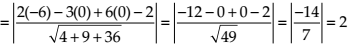error: Content is protected !!